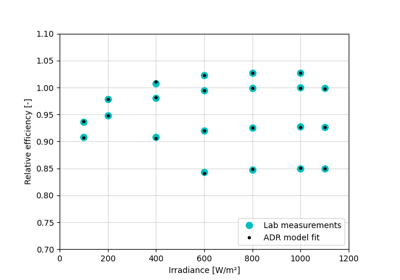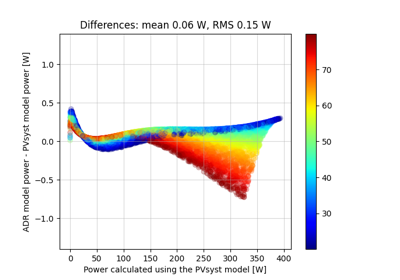Determine the parameters of the ADR module efficiency model by non-linear least-squares fit to lab or field measurements.

Parameters
• effective_irradiance (numeric, non-negative) – Effective irradiance incident on the PV module. [W/m^2]

• temp_cell (numeric) – PV module operating temperature. [°C]

• eta (numeric) – Efficiency of the PV module at the specified irradiance and temperature(s). [unitless] or [%]

• dict_output (boolean, optional) – When True (default), return the result as a dictionary; when False, return the result as a numpy array.

• kwargs – Optional keyword arguments passed to scipy.optimize.curve_fit. These kwargs can over-ride some options set within this function, which could be interesting for very advanced users.

Returns

popt (array or dict) – Optimal values for the parameters.

Notes

The best fits are obtained when the lab or field data include a wide range of both irradiance and temperature values. A minimal data set would consist of 6 operating points covering low, medium and high irradiance levels at two operating temperatures.

## Examples using `pvlib.pvarray.fit_pvefficiency_adr`#Obtaining ADR model parameters from IEC 61853 matrix measurements

Obtaining ADR model parameters from IEC 61853 matrix measurementsFast simulation using the ADR efficiency model starting from PVsyst parameters

Fast simulation using the ADR efficiency model starting from PVsyst parameters